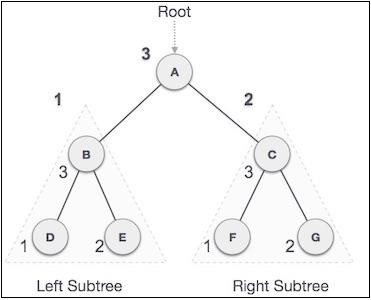# Post-order traversal in a Javascript Tree

JavascriptWeb DevelopmentFront End Technology

In this traversal method, the root node is visited last, hence the name. First, we traverse the left subtree, then the right subtree and finally the root node.We start from A, and following Post-order traversal, we first visit the left subtreeB. B is also traversed post-order. The process goes on until all the nodes are visited. The output of post-order traversal of this tree will be −

D → E → B → F → G → C → A

This is the algorithm we'll be implementing −

• Recursively traverse left subtree
• Recursively traverse right subtree
• Print the node's data

Let us look at how we'd implement it in our class.

postOrder() {
postOrderHelper(this.root);
}

Helper function −

## Example

function postOrderHelper(root) {
if (root !== null) {
postOrderHelper(root.left);
postOrderHelper(root.right);
console.log(root.data);
}
}

You can test this using −

## Example

let BST = new BinarySearchTree();
BST.insertRec(10);
BST.insertRec(15);
BST.insertRec(5);
BST.insertRec(50);
BST.insertRec(3);
BST.insertRec(7);
BST.insertRec(12);
BST.postOrder();

## Output

This will give the output −

3
7
5
12
50
15
10